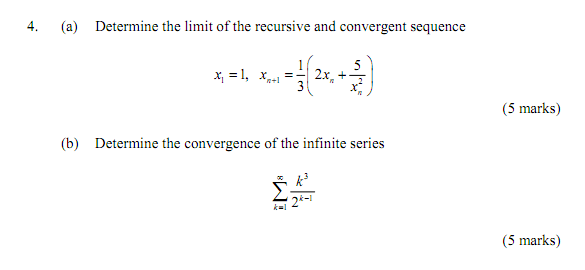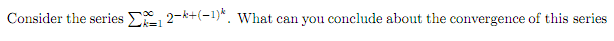Series and Sequence (convergence and divergence) - Singapore Forums by SGClub.comHome Photos Member List Register Mark Forums ReadHome » Series and Sequence (convergence and divergence)
 Why aren't you a member of SGClub.com yet?? » Join 130,000+ other members in chatting. » Make lots of new friends here. » Keep up-to-date with current events. » Participate in Club outings. » Download lots of Free Stuff! Registration just takes 2mins and is absolutely free so join our community today! I Want to Choose my Own Personal Nickname Now!12th November 2011, 10:26 PM #1 (permalink) Experienced SGClubberPosts: 1,634 Join Date: Dec 2009 Likes: 21 Liked 39 Times in 26 Posts Gender:Series and Sequence (convergence and divergence) Tweeti don't understand (a). (b) i used geometric series and got the answer "1". but the answer sheet gave me "0.5"... can someone tell me if i am the 1 who got it wrong or is the answer wrong?as for this question, i used ratio test and there are 2 possible answer as it approaches infinity due to the (-1)^n..... and as far as i can remember, if ratio test fails, doesn't it also mean that root test would fail? Sponsors: Last edited by KiLlErDeViL; 13th November 2011 at 12:52 AM.13th November 2011, 12:32 AM #2 (permalink) Experienced SGClubberPosts: 2,404 Join Date: Jul 2009 Likes: 0 Liked 211 Times in 174 Posts Gender:Re: Series and Sequence (convergence and divergence) Tweet I will settle 4(a) for you first. Two ways to find this converging limit: Method 1: As n tends towards infinity, X{n+1}=X{n} Hence we have X{n}=(1/3) [ 2* X{n}+ 5/( X{n}^2) ] Do some manipulation, you should end up getting (X{n})^3 =5 ie limit is simply cube root of 5 which is 1.7099759 Method 2: Interpret the recurrence relation as a curve with equation y=(1/3)[ 2x+ 5/(x^2) ]. Differentiate both sides wrt x and set dy/dx=0. You will also get the same answer. Note that so long as the starting term x1 is positive, when you cycle it through numerous iterations of the recurrence relation, all subsequent terms will also remain positive (you might want to graph the curve out to convince yourself) ; in the end it will ALWAYS converge to the minimum point of the curve y=(1/3)[ 2x+ 5/(x^2) ]. So it doesn't matter if X1=1 or X1=10000; ultimately the series will still converge to 1.7099759. (X1 is merely an insignificant token value) Hope this helps. Peace. __________________ White Group Mathematics Free A Level H2 Maths Resource Site Last edited by whitecorp; 13th November 2011 at 12:33 AM.13th November 2011, 12:37 AM #3 (permalink) Addicted SGClubberPosts: 362 Join Date: Jun 2007 Likes: 1 Liked 2 Times in 2 Posts Gender:Re: Series and Sequence (convergence and divergence) Tweet What course are you taking? For (a) it is a recurrence series. As for (b) the answer will not be 0.5 since when k=1, the term is 0.5 and the other term are non-negative. (Or ur telling me that ratio test should yield r=0.5 ? ) For qn 2, it is a GP but it will be easy to evaluate if you split the sum into the sum of having k divisible by 2 and k not divisible by 2.13th November 2011, 01:16 AM #4 (permalink) Experienced SGClubberPosts: 1,634 Join Date: Dec 2009 Likes: 21 Liked 39 Times in 26 Posts Gender:Re: Series and Sequence (convergence and divergence) Tweet Originally Posted by whitecorpI will settle 4(a) for you first. Two ways to find this converging limit: Method 1: As n tends towards infinity, X{n+1}=X{n} Hence we have X{n}=(1/3) [ 2* X{n}+ 5/( X{n}^2) ] Do some manipulation, you should end up getting (X{n})^3 =5 ie limit is simply cube root of 5 which is 1.7099759 Method 2: Interpret the recurrence relation as a curve with equation y=(1/3)[ 2x+ 5/(x^2) ]. Differentiate both sides wrt x and set dy/dx=0. You will also get the same answer. Note that so long as the starting term x1 is positive, when you cycle it through numerous iterations of the recurrence relation, all subsequent terms will also remain positive (you might want to graph the curve out to convince yourself) ; in the end it will ALWAYS converge to the minimum point of the curve y=(1/3)[ 2x+ 5/(x^2) ]. So it doesn't matter if X1=1 or X1=10000; ultimately the series will still converge to 1.7099759. (X1 is merely an insignificant token value) Hope this helps. Peace. correct me if i am wrong in understanding it Method 1: because n going towards infinity, even if n+1 = infinity + 1, where infinity + 1 is still infinity. tat's why you put "X{n+1}=X{n}" Method 2: other than this question which you use differentiation, am i allow to use this method to solve all other recursive questions? else, under what situation am i allow to use this method? so does that mean that in any question, if they give me X1= 1 or any positive value, it would just tell me that all subsequent terms are positive? and if they gave me X1=-1, all terms are negative? and so what happens if it is negative?13th November 2011, 01:25 AM #5 (permalink) Experienced SGClubberPosts: 1,634 Join Date: Dec 2009 Likes: 21 Liked 39 Times in 26 Posts Gender:Re: Series and Sequence (convergence and divergence) Tweet Originally Posted by IcystrikeWhat course are you taking? For (a) it is a recurrence series. As for (b) the answer will not be 0.5 since when k=1, the term is 0.5 and the other term are non-negative. (Or ur telling me that ratio test should yield r=0.5 ? ) For qn 2, it is a GP but it will be easy to evaluate if you split the sum into the sum of having k divisible by 2 and k not divisible by 2. electrical and electronics engineering (EEE). qn2: wad u mean by split? you mean like this? the 2 possible answers are as followed: L = 2^1 = 2 >1 , therefore diverge L = 2^-3 < 1, therefore converge so which is the answer? how am i suppose to choose from it? Last edited by KiLlErDeViL; 13th November 2011 at 02:27 AM.13th November 2011, 11:28 AM #6 (permalink) Addicted SGClubberPosts: 362 Join Date: Jun 2007 Likes: 1 Liked 2 Times in 2 Posts Gender:Re: Series and Sequence (convergence and divergence) Tweet For Method 1: We assume that the series is convergence. Therefore, x_{n}=x_{n+1} for some n (>=) N. For Method 2: It generally holds for "most" series since the series must not be monotone increasing or decreasing (lead to divergence) but a infinite points within (L+e,L-e). But the above statement is not a proof, I would recommend you to stick to method 1. For b, it is not a GP and are you using ratio test to proof its convergence or you are trying to evaluate the sum? For question 2, what I meant was to evaluate k=2m and k=2m-1 for m=1,2,3,... and splitting the sum. Last edited by Icystrike; 13th November 2011 at 11:32 AM.13th November 2011, 12:09 PM #7 (permalink) Experienced SGClubberPosts: 1,634 Join Date: Dec 2009 Likes: 21 Liked 39 Times in 26 Posts Gender:Re: Series and Sequence (convergence and divergence) Tweet Originally Posted by IcystrikeFor Method 1: We assume that the series is convergence. Therefore, x_{n}=x_{n+1} for some n (>=) N. For Method 2: It generally holds for "most" series since the series must not be monotone increasing or decreasing (lead to divergence) but a infinite points within (L+e,L-e). But the above statement is not a proof, I would recommend you to stick to method 1. For b, it is not a GP and are you using ratio test to proof its convergence or you are trying to evaluate the sum? For question 2, what I meant was to evaluate k=2m and k=2m-1 for m=1,2,3,... and splitting the sum. thx for the explanation. for b, what is GP? geometric? for b, i am trying to evaluate the sum, not just testing for its convergence qn 2, i haven't tried the method that u have mentioned, but by letting k=2m and k=2m-1, what test am i using? what kind of answer would it determine that its converges or diverges? e.g, L>1 = converge? L<1 = diverge?Thread ToolsShow Printable VersionEmail this Page Display ModesLinear ModeSwitch to Hybrid ModeSwitch to Threaded ModeSimilar Threads Thread Thread Starter Forum Replies Last Post maverick Automobile Forum 18 23rd July 2011 08:53 PM Tit Health & Fitness 21 28th September 2010 10:58 PM black- School Life 5 20th July 2010 04:55 PM prolohz General Merchandise 0 29th November 2008 12:54 AMFeatured Photos by marisoljames322 · · · Member Galleries 20359 photos13619 comments by marisoljames322 · · · Member Galleries 20359 photos13619 comments by pdsubbu · · · Member Galleries 20359 photos13619 comments by Vikas Dhar · · · Photography 35 photos36 comments by aaudreygan · · · Guy Photos 1581 photos946 comments by aaudreygan · · · Girl Photos 4351 photos28606 comments by a8paris · · · Guy Photos 1581 photos946 comments by a8paris · · · Guy Photos 1581 photos946 comments by aaricia · · · Girl Photos 4351 photos28606 comments by aaricia · · · Girl Photos 4351 photos28606 commentsCopyright© 2004-2013 SGClub.com. All rights reserved.